Killer Tips to Write Harder Measures - Excelerator BI

# Killer Tips to Write Harder Measures

Level: Intermediate

## Background

I was reading the questions at http://community.powerbi.com today and saw an interesting question.  In short the OP wanted to know if there was a way to identify (via a measure) if the row in a table had a colour =”Blue”, but also to check that there were no other rows with the same ID.  In other words, the row had to be colour=”Blue” and also there had to be no other rows for the same ID in the table.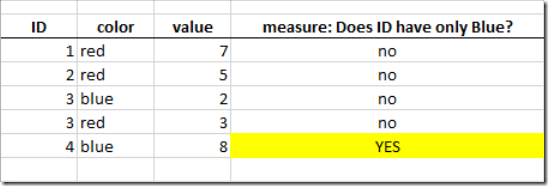Here is the original post

https://community.powerbi.com/t5/Desktop/Can-a-Measure-sum-other-rows-besides-itself/m-p/288285#M126830

The OP was pretty sure it couldn’t be done with a measure. I was pretty sure it could so I set about creating a measure to demonstrate.## How I Write Measures (tricky ones anyway)

This blog post is about “how” I solved this problem.  I have blogged about my process previously here, although that was a calculated column.

• I knew that it was possible to filter the entire underlying table based on the ID in the row.
• I knew there was a CONTAINS function, although I had never used it before – it seemed like a likely candidate

## Killer Tip 1: Create Good Test Data

The first thing I did was to replicate the test data shown above.  As I have mentioned many times, good quality test data is essential to getting a quick correct answer to your problem.  The data from the OP looked pretty good as it had covered the relevant scenarios (1 ID had just blue, 1 ID had blue and red, several IDs had no blue – this is good test data).

## Killer Tip 2: Set Up a Table (or Matrix) to See the Results

After loading the data in Power BI Desktop, I immediately set up a table on the report canvas.  This is fundamental to good measure writing and testing.  If you can’t “see” how your measure reacts to your test data, you will struggle to make progress.  So I simply loaded a table like this.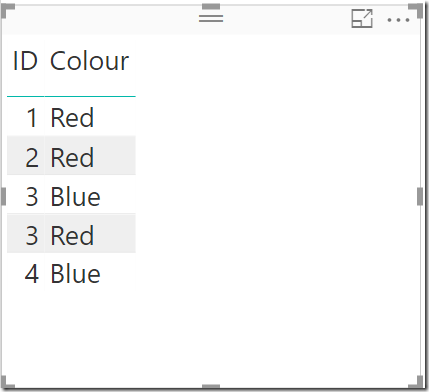## Killer Tip 3: Break the Problem Down

Don’t try to solve world peace on your first attempt. Break your DAX problem down into manageable pieces and solve a little bit of the problem as you take each step forward.  Here is what I did.

### First Test Measure

The first thing I did was to write the FILTER function and used it inside a CALCULATE as you can see below.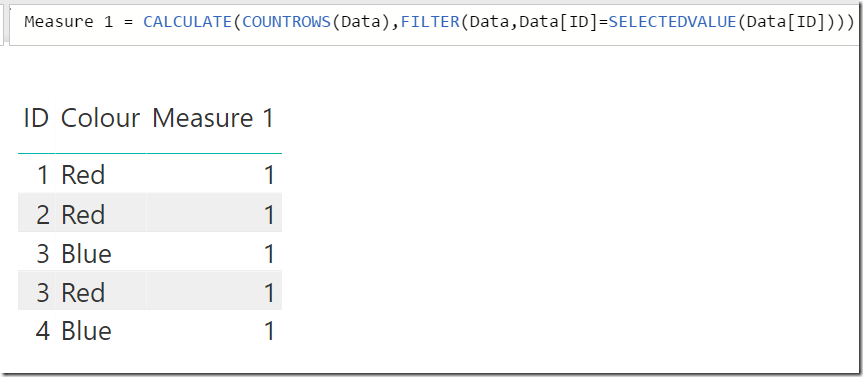At first sight I was a bit surprised that I got the answer 1 all the way down the table – I was expecting 2 for the rows with ID 3.  But then I quickly realised what was happening. I learnt this when I attended Marco Russo’s Mastering DAX class.  In short, if a table doesn’t contain a primary key, then it will use the concatenation of all columns as a substitute primary key (quite complex and out of scope for this blog).  Anyway, this clearly demonstrates my point about breaking the problem into pieces.  Before moving on, I could “see” this formula was wrong.

### Second Test Measure

I knew exactly how to fix the problem, by adding ALL around the table.  Here was my second measure.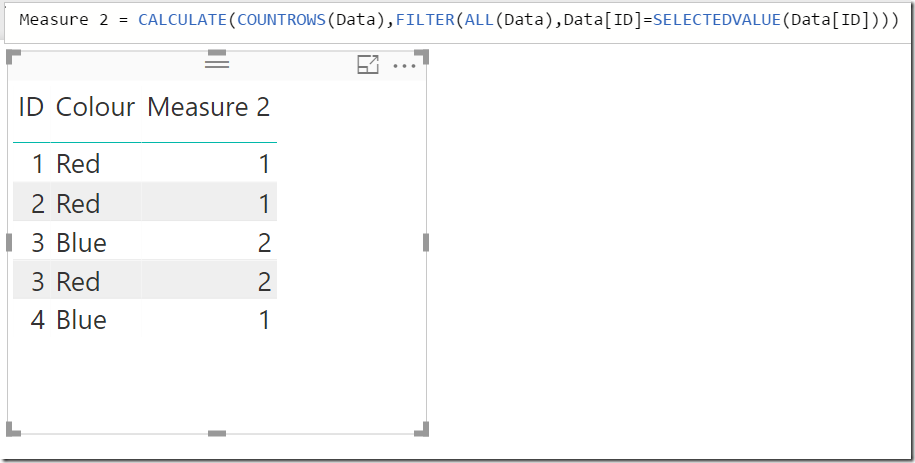Now the ALL function around the table removes the filter applied by the concatenation of ID and Colour before reapplying a filter just on the ID column.  Now it is clear that my filter is working correctly.

### Third Test Measure

Now I had the FILTER working, it was time to check out the CONTAINS function.

## Killer Tip 4:  Read the Intellisense

As I wrote the measure, I just used Intellisense to guide me (no online documentation needed here).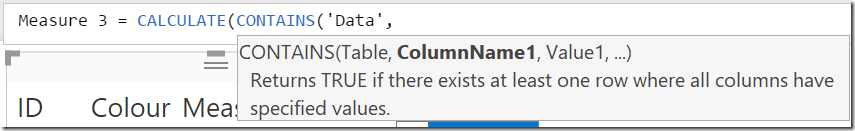See above how Intellisense provided me the syntax as well as a text description of how the function works.  I knew immediately this was the right function.  I replaced the COUNTROWS interim test portion with the CONTAINS function as shown blow.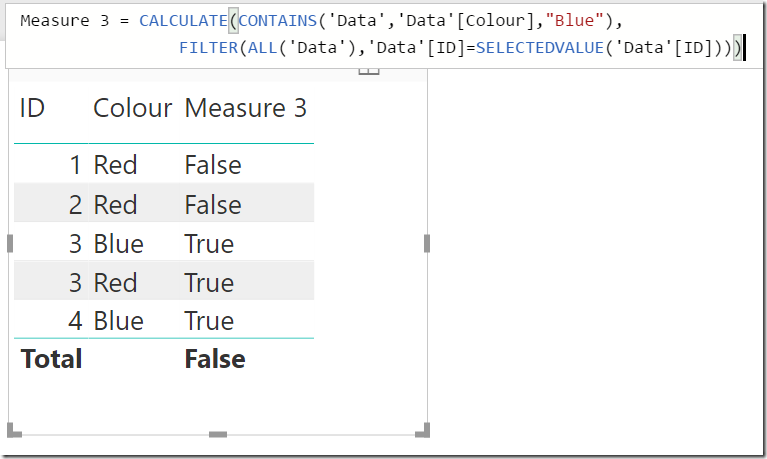Measure 3 was now telling me if the ID had at least 1 row where colour = “blue”.## Final Measure

The last thing I needed to do was modify the function so that it returned TRUE if and only if the current row was Blue and there were no other rows for that ID.  That seemed pretty straight forward.  If there is only 1 row for that ID, and if the colour of the ID was blue, then I was done.  Here is my final measure.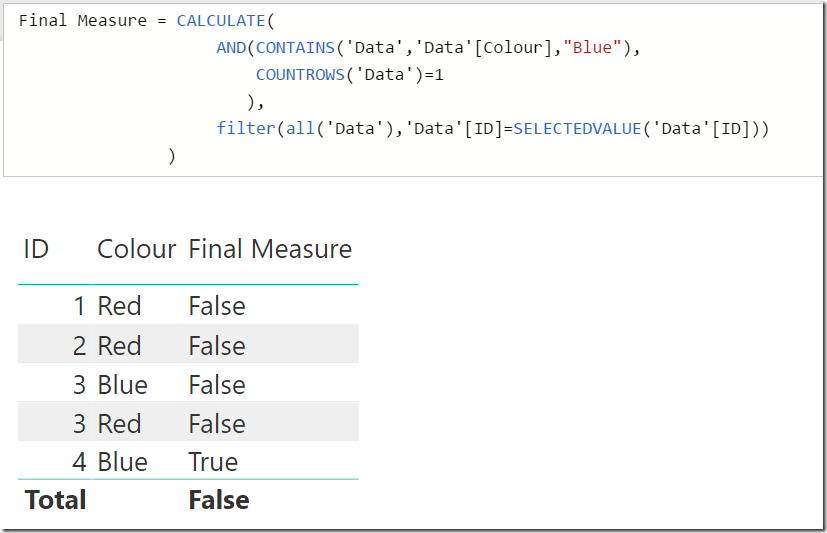I just wrapped the CONTAINS statement inside and AND, and added a second condition to see if there was only 1 row in the table.

I hope this walked through example and killer tips help you become more self sufficient with your DAX.  Here is my workbook. only blue

### 13 thoughts on “Killer Tips to Write Harder Measures”

1.How would you display the results in a graph? For example, only one of the 5 rows returns the value of “True” based on the measures you’ve written, but when trying to graphically display it, I cannot get the values from the final measures column to display on the graph. If you ran this for several thousand rows, you might want to know how many unique id’s with only “Blue” there are. It seems as if the results of the measures are not treated as data and therefore are not able to be displayed in that manner?

Please forgive me if this is a dumb question, I’m new to BI.

1.What sort of chart would you want to use? There are no numbers in this table so there is nothing to display. I guess you could do a count of colour or something like that

1.My first thought would be the number of “True” results so yes a count would work. I was thinking of charting (really any kind of chart, but just a bar graph would be fine) the total number of “True” vs. “False” results. I made an assumption that this would work automatically with a filter without having to count or otherwise calculate the number of those different results. In Excel, a simple CountIf would get me the figures and I’d have figured it out right away, but in BI, I’m a total newb as to the best/most efficient way to use the result of the measure to do it.

1.I didn’t explain that very well. Here’s the thing, I can select a chart and display that there are two “Blue” and three “Red” colours but I cannot get that same bar graph to display that there are four “False” results and one “True” by switching up the columns I want to filter. Red and Blue are not numeric in the same manner as True/False. That’s where I was stuck.

1.That’s correct. It’s because these are measures and not columns. You can’t put measures on a column, row or axis. If you write an equivalent calculated column then it will do what you want.

2.I also completed the Mastering Dax class and came up with a version similar to what you provided.
Final = VAR IDCount = CALCULATE(COUNTROWS(Data), FILTER(ALL(Data), Data[ID] = MAX(Data[ID])))
VAR ColourCount = COUNTROWS(FILTER(Data, Data[Colour] = “Blue”))
Return IF(AND(IDCount=1,ColourCount=1),”Yes”,”No”)

3.@Matt – If you are happy with displaying TRUE / FALSE
This will work
mStatus
=NOT(IF(HASONEVALUE(D[Color]),IF(VALUES(D[Color])=”BLUE”,CALCULATE(COUNT(D[ID]),ALL(D[Color])),0),BLANK())-1)

4.Itumeleng Nomlomo

Thanks Matt. Why did you opt for a measure instead of a calculated column? Considering that the outcome is specifically limited to TRUE or FALSE, I would have considered using a calculated column.

1.I selected a measure for the sole reason that the original post specifically asked for a measure with the statement “I don’t think it is possible”. Even though the result is true/false, personally I would only use a calc column if I wanted to use the true/false in a slicer or rows in a matrix.

5.Hi Matt,
A fun albeit somewhat less understandable way to do the same thing is this measure:

My one and only blue =
COUNTROWS (
CALCULATETABLE ( VALUES ( ‘Data'[colour] ), ALLEXCEPT ( ‘Data’, ‘Data'[ID] ) )
)
* COUNTROWS (
CALCULATETABLE (
VALUES ( ‘Data'[colour] ),
KEEPFILTERS ( ‘Data'[colour] = “Blue” )
)
)
= 1

For maintenance reasons people are probably better off using your version.

Best regards Jes

1.I love the way you think Jes 🙂

1.🙂 Glad you like my thinking Matt. The measure was meant as an alternative solution different from yours. The multiplication in the measure probably makes it difficult for the query optimizer to find an optimal query plan so I’ll paste my original solution for comparison to yours. It uses the same logic as your measure in the article so there isn’t much new added. (Hence my alternative measure 🙂 )

My one and only blue – plain logic :=
COUNTROWS (
CALCULATETABLE ( VALUES ( ‘Data'[Colour] ), ALLEXCEPT ( ‘Data’, ‘Data'[ID] ) )
)
= 1
&& NOT ( ISEMPTY ( FILTER ( VALUES ( ‘Data'[Colour] ), ‘Data'[Colour] = “Blue” ) ) )

6.Nice post Matt. One more concrete foundation on writing DAX formulas from you.
Building a measure incrementally and testing it at every step is a wonderful idea.
It ensures that the final formula works correctly. And in case it doesn’t by any chance, it is easy to step back and check where the error is occurring.

Scroll to Top